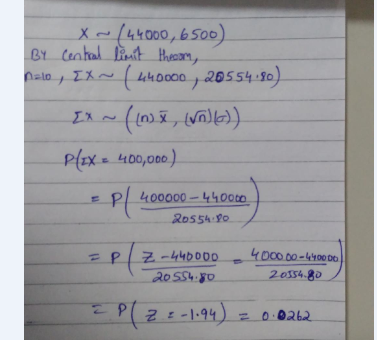##### Question

In: Statistics and Probability

# Salaries for teachers in a particular elementary school district are normally distributed with a mean of...

Salaries for teachers in a particular elementary school district are normally distributed with a mean of $44,000 and a standard deviation of$6,500. We randomly survey ten teachers from that district.
1.Find the probability that the teachers earn a total of over $400,000 2.If we surveyed 70 teachers instead of ten, graphically, how would that change the distribution in part d? 3.If each of the 70 teachers received a$3,000 raise, graphically, how would that change the distribution in part b?

## Solutions

##### Expert Solution1.0.0262

2. Probability increases as sample size increases. So sampling 70 teachers instead of ten would cause the distribution to be more spread out. It would be a more symmetrical normal curve.

##### Salaries for teachers in a particular elementary school district are normally distributed with a mean of...
Salaries for teachers in a particular elementary school district are normally distributed with a mean of $46,000 and a standard deviation of$4,900. We randomly survey ten teachers from that district. (Round your answers to the nearest dollar.) (a) Find the 90th percentile for an individual teacher's salary. (b) Find the 90th percentile for the average teacher's salary.
##### Salaries for teachers in a particular elementary school district are normally distributed with a mean of...
Salaries for teachers in a particular elementary school district are normally distributed with a mean of $46,000 and a standard deviation of$4,500. We randomly survey ten teachers from that district. (Round your answers to the nearest dollar.) A) Find the 90th percentile for an individual teacher's salary. B)Find the 90th percentile for the average teacher's salary.
##### Salaries for teachers in a particular elementary school district are normally distributed with a mean of...
Salaries for teachers in a particular elementary school district are normally distributed with a mean of $42,000 and a standard deviation of$5,700. We randomly survey ten teachers from that district. (Round your answers to the nearest dollar.) (a) Find the 90th percentile for an individual teacher's salary. $= (b) Find the 90th percentile for the average teacher's salary.$ =